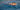Analysis

August 30, 2018 5 minute read

## 4 Things You Should Stop Doing in SQL and Start Doing in PythonData Scientist

As analysts, we know that each question we ask of our data can be answered with multiple potential languages and toolkits. Each language has its strengths and we've often pondered the distinctions. We've previously examined Group By, window functions and a general framework for thinking in SQL and Python.

While our position has generally been language agnostic, there are some actions in SQL that are simply more efficient in Python. In this post, we'll share 4 time-saving examples where Python is far superior than SQL for exploring and analyzing your data set.

### Descriptive stats

Let's say you're exploring a new dataset. There're lots of different ways to statistically describe the data and get a feel for it. For example:

• Count
• Mean
• Standard Deviation
• Minimum Value
• 25th Quartile distribution
• 50th Quartile distribution(Median)
• 75th Quartile distribution
• Maximum Value

To gather this information in SQL, you'd write something like this:

``````SELECT ROUND(MIN(first_class_rev)::numeric, 2) AS min,
ROUND(PERCENTILE_CONT(0.25) WITHIN
GROUP (ORDER BY first_class_rev)::numeric, 2) AS pc25,
ROUND(PERCENTILE_CONT(0.50) WITHIN
GROUP (ORDER BY first_class_rev)::numeric, 2) AS median,
ROUND(AVG(first_class_rev)::numeric, 2) AS mean,
ROUND(PERCENTILE_CONT(0.75) WITHIN
GROUP (ORDER BY first_class_rev)::numeric, 2) AS pc75,
ROUND(MAX(first_class_rev)::numeric, 2) AS max
FROM tutorial.flight_revenue
``````

But perhaps you don't want to type out all that code. You can use pandas' DataFrame.describe() function to return basic descriptive statistics for the underlying dataset.

``````datasets['Python Input'].describe().round(1)
``````

Check out this report and clone it to explore descriptive statistics on your own.

### Moving Averages

Now let's say you'd like to calculate moving averages to get a clear average on continuously changing input. Moving averages serve to smooth out sharp dips and spikes in your data, so that long term trends can become more observable.

In SQL, you could write out a query like this one:

``````WITH input AS (
SELECT
COUNT(1) AS trips,
DATE_TRUNC('day',start_date) AS date
FROM modeanalytics.sf_bike_share_trip
GROUP BY 2
)

SELECT
trips,
AVG(trips) over (order BY date rows between 13 preceding AND current row) AS mvg_avg, -- Window function to calculate 14-day moving average of ride share trips
SUM(trips) over (order BY date rows unbounded preceding) AS running_total_trips, -- Window function to calculate the running total number of ride share trips
lag(trips,7) over (order BY date) AS num_trips_previous_day, -- Window function to grab the number of trips on the previous day
trips - lag(trips,7) over (order BY date) AS wow_difference,
(trips - lag(trips,7) over (order BY date))/lag(trips,7) over (order BY date)::DECIMAL(18,2) AS wow_percent_change, -- Window function to calculate the week-over-week percent increase in trips
date
FROM input
ORDER BY date;
``````

In Python, you could quickly achieve the same, two-week moving average with the following code:

``````import numpy as np
import pandas as pd
import matplotlib.pyplot as plt
df = datasets["Trips - Python Window"]
df["mvg_avg"] = df.trips.rolling(14).mean()
``````

In addition, Python allows you to take the visualization even further. Check out this report that gives additional options for visualizing moving averages in Python. You'll see comments to walk you through perfecting your report in Python.

### Pivot

To re-arrange data and pivot for charting or a presentation-ready format, you'll want to take a few steps in SQL. For this example, we're going to pivot rows to columns from the College Football players dataset in the Mode Public Warehouse (view the data here).

We'll first aggregate the players from each year and conference to prepare the data.

``````SELECT teams.conference AS conference,
players.year,
COUNT(1) AS players
FROM benn.college_football_players players
JOIN benn.college_football_teams teams
ON teams.school_name = players.school_name
GROUP BY 1,2
ORDER BY 1,2
``````

Next to transform the data, we'll nest the query in a subquery.

``````SELECT *
FROM (
SELECT teams.conference AS conference,
players.year,
COUNT(1) AS players
FROM benn.college_football_players players
JOIN benn.college_football_teams teams
ON teams.school_name = players.school_name
GROUP BY 1,2
) sub
``````

Then, you'd want to break out the results into columns for each year. You can create separate columns with each item in the SELECT statement.

``````SELECT conference,
SUM(CASE WHEN year = 'FR' THEN players ELSE NULL END) AS fr,
SUM(CASE WHEN year = 'SO' THEN players ELSE NULL END) AS so,
SUM(CASE WHEN year = 'JR' THEN players ELSE NULL END) AS jr,
SUM(CASE WHEN year = 'SR' THEN players ELSE NULL END) AS sr
FROM (
SELECT teams.conference AS conference,
players.year,
COUNT(1) AS players
FROM benn.college_football_players players
JOIN benn.college_football_teams teams
ON teams.school_name = players.school_name
GROUP BY 1,2
) sub
GROUP BY 1
ORDER BY 1
``````

While this gives you a great deal of control over your query and process, there is an easier way to achieve this in Python. Once you SELECT from the College Football Players, from year and conference, you can jump into the Notebook and run DataFrame.pivot. This allows you to reshape data based on column values, so that you can rearrange result sets.

``````DataFrame.pivot
``````

To explore how we created these pivots, check out this Mode report.

### Self Join

There are plenty of instances where you might want to join a table to itself. To create a join, you'd first want to write this query that gives different reference names to the same table.

``````SELECT DISTINCT japan_investments.company_name,
FROM tutorial.crunchbase_investments_part1 japan_investments
JOIN tutorial.crunchbase_investments_part1 gb_investments
ON japan_investments.company_name = gb_investments.company_name
AND gb_investments.investor_country_code = 'GBR'
AND gb_investments.funded_at > japan_investments.funded_at
WHERE japan_investments.investor_country_code = 'JPN'
ORDER BY 1
``````

In pandas, we can achieve this via

``````d = pd.DataFrame(['A','B','C'], columns = ['Column_1'])
d['Column_2'] = [1,2,1]
d.join(d.drop('Column_2', 1), on='Column_2', rsuffix='.1')
``````

Want to play around with self joins on your own? Clone this report into your personal Mode space and our comments will guide you.

There are always many ways to achieve the same result, but these tips will help you work smarter, not harder.